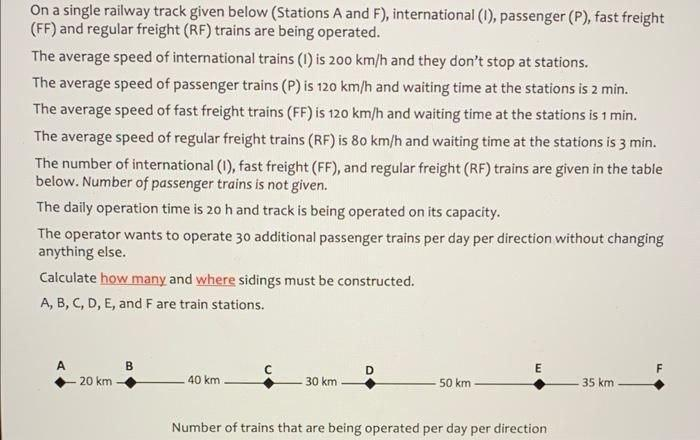Home / Expert Answers / Civil Engineering / railway-engineering-i-2-ff-7-rf-4-on-a-single-railway-track-given-below-stations-a-a-pa396

# (Solved): Railway engineering I: 2 ; FF: 7 ; RF: 4 On a single railway track given below (Stations $$A$$ a ...

Railway engineering I: 2 ; FF: 7 ; RF: 4On a single railway track given below (Stations $$A$$ and $$F$$ ), international $$(1)$$, passenger $$(P)$$, fast freight (FF) and regular freight (RF) trains are being operated. The average speed of international trains (I) is $$200 \mathrm{~km} / \mathrm{h}$$ and they don't stop at stations. The average speed of passenger trains $$(P)$$ is $$120 \mathrm{~km} / \mathrm{h}$$ and waiting time at the stations is 2 min. The average speed of fast freight trains (FF) is $$120 \mathrm{~km} / \mathrm{h}$$ and waiting time at the stations is $$1 \mathrm{~min}$$. The average speed of regular freight trains (RF) is $$80 \mathrm{~km} / \mathrm{h}$$ and waiting time at the stations is $$3 \mathrm{~min}$$. The number of international (I), fast freight (FF), and regular freight (RF) trains are given in the table below. Number of passenger trains is not given. The daily operation time is $$20 \mathrm{~h}$$ and track is being operated on its capacity. The operator wants to operate 30 additional passenger trains per day per direction without changing anything else. Calculate how many and where sidings must be constructed. $$A, B, C, D, E$$, and $$F$$ are train stations.

We have an Answer from Expert